Sliced PolyominoesThe removal of part of a polyomino by various types of diagoinal cuts or by adding squares to diagonally cut squares, dominoes or trominoes has been looked at in some detail. Most sets produced sre not very interesting but a few give some interesting figures. Click on the links (yellow rectangles) below to see samples. These sets are described in more detail below.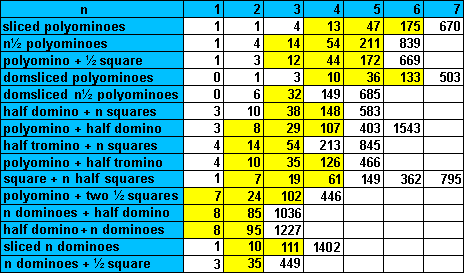If we consider one-sided sets then we get the following numbers of pieces. The half domino and half tromino sets are omitted as they have no symmetrical pieces.Other variations are possible as shown in the links in the table below.If we were to remove half a square with a single diagonal cut then we get 13 sliced tetrominoes of area 3½ unit squares. With this set we can make two 3x7 rectangles with one piece omitted or a 7x7 square with one piece used twice (or the second piece could be counted as a hole).It is also possible to make a 'sliced rectangle' with the full set.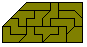The one-sided set consists of the 25 pieces shown in the constructions below. No rectangle is possible unless we use one piece twice as in the figure at the right below.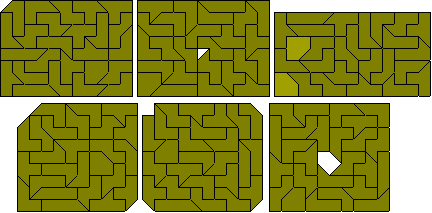A number of sliced rectangles can be made with this set.If we allow an original join to be removed then we get one extra piece and this set of 14 pieces can make a square as well as a number of other symmetrical figures. This set has been referred to as the 3½-ominoes.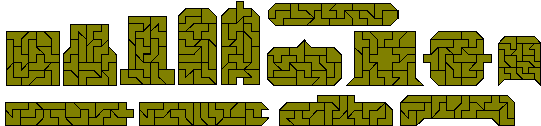It is also possible to make a number of congruent pairs with the set.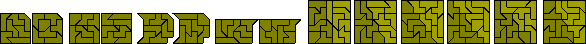We can also make four fold replicas of three of the pieces with the piece replicated used three times. The remaining pieces cannot be thus replicated as it is not possible to fill a 45º corner. It might also be possible to use the set to form a duplication and a triplication of one of the pieces but this is probably only possible for the piece shown at the right below. The piece omitted cannot be the piece replicated.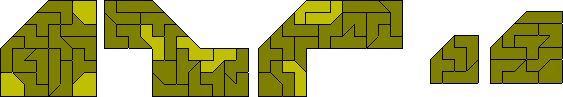If we consider the one-sided set then we get the following 27 pieces which are shown forming the only two possible sliced rectangles. Also included are soem examples of the similar hole problem for this set.Roel Huisman has some problems based on this and other polyforms.

There are 47 sliced pentominoes and 54 4½-ominoes with seven extra pieces. The sliced pentominoes can make a number of rectangles if one piece is used twice.The 47 pieces can also make a number of 'sliced rectangles'.It is also possible to make a similar hole construction with this set with one piece used twice.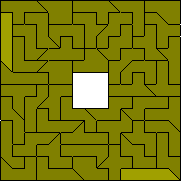The 54 4½-ominoes can make a 9x27 rectangle as shown here where the seven extra pieces are coloured paler than the rest.An improvement on this would be to form three 9x9 squares as shown here.Other figures formed with this set are shown below including the four possible sliced rectangles.There are many variations on the 9x27 rectangle the first set here is by Roel Huisman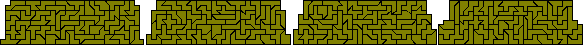It is also possible to form two congruent shapes with this set. The second solution provides a new way of forming the 9x27 rectangle.There are many other possible symmetrical figures to be made with this set some of which are shown below.Roel Huisman has found sets of six and nine congruent shapes with this set.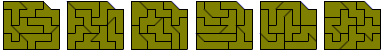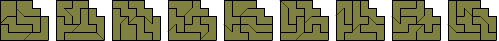If we consider the one-sided set of sliced pentominoes then there are 92 pieces with a total area of 414 squares.This gives three possible rectangles which can be made - 6x69, 9x46 and 18x23 all of which are possible as seen below where the last two are formed from two 9x23 rectangles. For sliced rectangles we have the 12 possibilities listed below. The first nine of these are shown in the diagram below but the last three may not be possible owing to the difficulty of forming the sliced edge.For the one-sided 4½-ominoes there are 106 pieces. Only a 9x53 rectangle is possible which is shown below together with four of the six sliced rectangles with the required area. The other two may not be possible..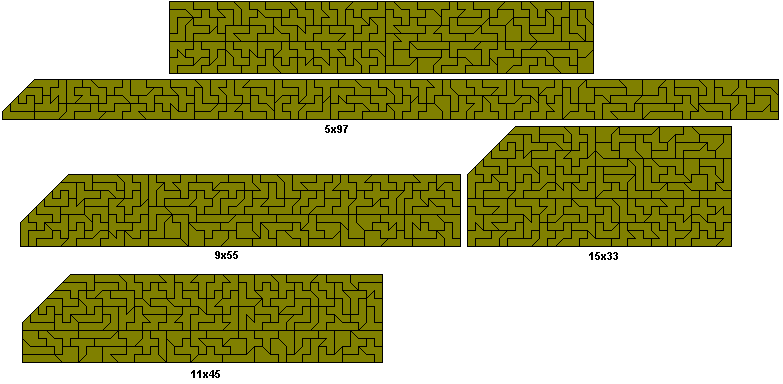If we omit the one piece with a hole then we get 210 5½-ominoes which Roel Huisman has packed into the series of rectangles shown here.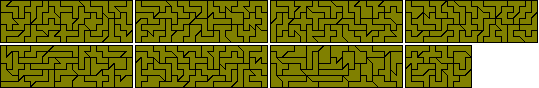Roel Huisman has found the 36 pieces obtained by removing half a domino from a pentomino. Roel calls them domslicedpentominoes and a large numbed of constructions are possible with the set as can be seen here.

If we allow pentominoes to be cut so that an internal join is removed then we get an extra two pieces (shown lighter in the first diagram below). This set cannot make any rectangle.Roel has also looked at constructions made with two sets of domsliced tetrominoes.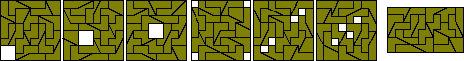We could also look at the set of one-sided domslicedtetrominoes which gives the same number of pieces but ensures that for each pair of pieces both of the mirror pair is used. Rectangles are certainly possible as shown here where the left hand pair of rectangles give solutions to the 5x12 and 6x10 rectangles and the right hand figure shows a 4x15 rectangle. The first four constructions below based on an 8x8 square are all made of two congruent parts.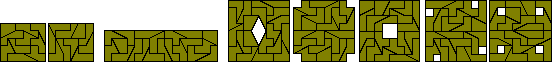While no rectangle can be made with a single set of pieces, a variety of symmetrical figures can be made with a single set of pieces. Also the one sided set can make a number of sliced rectangles.There are many other possible variations of adding/removing half squares/dominoes from the polyominoes. The following table gives some numbers of pieces for various sets. (Peter Esser has an excellent solver which works with all these sets and it can be downloaded from http://members.tripod.de/polyforms/psolver.zip.Roel Huismann has found a way of forming six congruent shapes with 174 of the 175 sliced hexominoes and eight congruent shapes using one piece twice.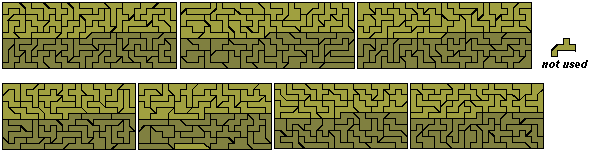A number of sliced rectangles are possible with this set.